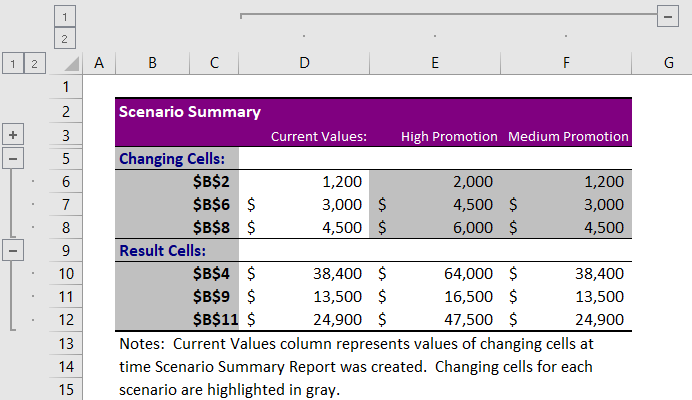# Scenario Manager in ExcelArticle byJeevan A Y## What Is A Scenario Manager In Excel?

Scenario Manager in Excel is a What-If Analysis tool that enables users to create different scenarios by changing the variables. For example, it allows us to change the input cells or variable cells and create different scenarios.

For example, a business analyst has to prepare a budget based on the best of his knowledge. However, there will be several variable factors that may change, so he needs to consider all the best and worst scenarios as it impacts the final output value. Scenario Manager will help us create those scenarios in Excel.

###### Key Takeaways
• The scenario Manager in Excel assists us in making different scenarios based on changes in variable inputs and gives us a rundown of all the created scenarios.
• It is available in Data-> What-If-Analysis-> Scenario Manager…
• It helps create various alternative results according to the changes in various variable values.
• It is a What-If Analysis tool that helps users identify the best marketing plan with given alternative values.
• The Scenario Manager creates a summary of all the scenarios and give the user the best chance to compare and make decisions.

### How To Use Scenario Manager In Excel? (With Steps)

Scenario Manager is quite simple and easy to understand. Let us look at a simple example to understand it better.

Mr. Anderson is a working professional. He also owns a house, and thus, he gets a monthly income. The following table clearly shows his monthly income and expenses.

• In the table, the cell range A2:B3 shows his income sources (as salary and rental). In cell B4, we can see the total income.
• His monthly expenses are listed in the cell range A7:B14. The total expense is displayed in cell B15.
• In cell B17, we have the total savings. This value is obtained by applying the formula total income (B4) minus total expenses (B14).

Now, Mr. Anderson wants to know how his savings amount will change when there is an increase in expenses. Let us look at the following scenarios.

Scenario 1

• Home loan (cell B9) is increased by \$1000
• Traveling cost (cell B11) is increased by \$200

Scenario 2

• Home loan (cell B9) is increased by \$1000
• Car loan (cell B8) is increased by \$500
• Traveling cost (cell B11) is increased by \$400
• Other expenses (cell B14) are increased by \$300.

The steps to find the potential savings amount for Mr.Anderson in both scenarios are as follows:

Step 1: First, go to the Data tab.

Click on the What-If Analysis Excel drop-down list and select Scenario Manager

Step 2: The Scenario Manager window appears. Click on the Add button.

Step 3: Then, in the Add Scenario window, we can name the scenarios in the Scenario name: box. In our example, let us name the first scenario as Scenario 1.

Step 4: Next, in the first scenario, we should change the home loan (cell B9) and traveling cost (cell B11).

So, choose these two cells in Changing cells: box. Click OK.

Step 5: The Scenario Values dialogue box pops up. Here, we need to change the values of the cells, i.e., home loan and traveling cost.

Step 6: So now, we have added the first scenario.

Similarly, we should click on the Add button to add the second scenario.

Step 7: Let us name the second scenario as Scenario 2.

Step 8: In this scenario, we need to change the values of Home loan (cell B9), Car loan (cell B8), Traveling cost (cell B11), and Other expenses (cell B14).

Therefore, we should select the 4 cells.

Click OK

Step 9: In the Scenario Values window, we should change the values of the cells.

Click OK.

Step 10: In the window, Scenario Manager, we can see the list of scenarios.

Double-click on any of the scenarios to see its impact. Otherwise, choose the scenario and click the Show button to see the impact.

Click on the Summary tab to summarize all the scenarios we have created.

Next, excel will prompt us to select the result cell that the altering variables will influence in the Scenario Summary window.

We have chosen cell B17 because the changes will impact this cell.

Click OK. Excel returns all the scenarios as shown in the image below.

Clearly, we can see the results in 3 scenarios. The first value is the original with a total savings of \$3600.

Mr. Anderson can save \$1,600 and \$1,400 from Scenario 1 and Scenario 2 respectively.

### Examples

Let us learn about scenario manager in excel with the following examples.

#### Example #1: Choose the Best Marketing Plan

Mr. Joe is a marketing manager and creates various discount offers to sell his products. For example, he is planning to sell 1500 units of perfume bottles at a \$3 discount which gives him a revenue of \$27,000.

Formula Explanation:

In cell B5, we have applied the following formula to calculate the total sales.

Units * Unit Price = B2 * B3 returns Gross Sales.

Likewise, Units * Discount = B2 * B4 gives the total Discount Value.

To obtain Total Sales, the formula is Gross Sales – Discount Value = Total Sales.

Mr. Joe has 3 scenarios with him:

• Risk: Perfume Units = 2000 and Discount = \$15
• Low Risk: Perfume Units = 1800 and Discount = \$7
• Medium Risk: Perfume Units = 1500 and Discount = \$8

When units and discounts change, Mr. Joe wants to know the effect on total sales and make a decision based on the best sales-yielding scenario.

Let us create scenarios for Mr. Joe by following the steps and learn how to use Scenario Manager in Excel.

Step 1: To begin with, open Scenario Manager under the Data tab.

Step 2: Let us name the first scenario as Risk.

Step 3: Then, select the cells B2 and B4 in the Changing cells: box and click OK.

Step 4: Now, change the B2 cell value to 1000 and the B4 cell value to 15.

Step 5: Next, click on Add to create another scenario. Let us name the second scenario as Low Risk.

Step 6: Since the changing cells are the same, click OK.

Then, change B2 cell value to 1800 and B4 to 7 in the Scenario Values window.

Step 7: Click Add to create the last scenario. Give a name for this scenario as Medium Risk and click OK.

Step 8: The Scenario Values window pops up. Here, change the B2 cell value to 1500 and the B4 cell value to 8.

Step 9: Now, all the scenarios are created. Click OK, and Excel lists the created scenarios in the Scenario Manager window.

As we can see in the above image, we have 3 scenarios, i.e., Risk, Low Risk, and Medium Risk.

Step 10: To see each scenario’s impact, select the scenario and click on the Show button to see the scenario result.

Likewise, we can choose each scenario and view the results individually. However, the scenario manager can easily compare the current value with the results.

Now, click on the Summary tab to compare each scenario with another.

Step 11: Now, we need to choose the result cell which will be impacted by the changing cells, i.e., cell B5.

Click on OK, and we will have a summary of each scenario.

From the table, it is clear that the Risk scenario will provide sales of \$15,000; Low Risk scenario will provide sales of \$41,400, and Medium Risk scenario will provide sales of \$33,000.

Mr. Joe can choose the scenario Low Risk, as this offers sales of \$41,400.

Thus, from this example of Scenario Manager in Excel, we understood how to create scenarios and compare the results.

#### Example #2

ABC Co. has prepared the following profit estimation.

ABC Co. has recorded that the operating variable costs change when the quantity increases.

Whenever the values change, ABC Co. wants to know the scenarios for profit. Following are the scenarios:

High Promotion: Quantity = 2000, Operating Cost = \$4500, Variable Cost = \$6000

Medium Promotion: Quantity = 1200, Operating Cost = \$3000, Variable Cost = \$4500

Let us create scenarios for ABC Co. using scenario manager in excel.

Step 1: First, open Scenario Manager… under the Data tab.

Step 2: The Add Scenario window appears. Now, let us type the first scenario as High Promotion.

Step 3: Next, choose the cells B3, B6, and B8 under changing cells. Click OK.

Step 4: Then, change the values as per the scenarios.

Step 5: Click on the Add button to create another scenario. Give a name to this scenario as Medium Promotion. The changing cells will be the same.

Step 6: Click OK and change the values of the cells in the Scenario Values window.

Step 7: Click OK. We will have all the scenarios.

Step 8: To view the result of these scenarios, select the scenario and click on the Show button.

When we choose the High Promotion scenario, the Total Profit increases to \$47,500.

Similarly, select Medium Promotion and click on Show to see this scenario result.

Medium Promotion has profit of \$24,900 but the costs decrease.

Clearly, we learned how to create scenarios using scenario manager in excel.

Create Summary Of All Scenarios

After creating the scenarios, it is tiresome to see the results one by one.  To avoid this difficulty, we can create a summary of all the scenarios in the new worksheet and compare them and make the best choice from the scenarios.

Once all the scenarios are created in the Scenario Manager window, we need to click on the Summary tab.

After clicking on the summary tab, Excel will ask us to choose the cells (those with results) where the output will change. In this case, choose cells B4, B9, and B11.

Click OK.

Now we have a scenario summary, as shown in the image below.

From the above image, it is clear that High Promotion gives us a maximum profit of \$47,500 even though costs are high.

This way, by creating a scenario summary, we can compare each scenario and make the best choice.

### Important Things To Note

• The Scenario Manager can choose a maximum of 32 cells to change values.
• To perform scenario management, we should have our table ready, i.e., all the original calculations and the necessary formulas should have been applied.
• The Scenario Manager shows only the cell addresses while entering new values, and one should be aware of what value is present in the particular cell address.
• The Show option of Scenario Manager will show only the impact of the selected scenario. While using the summary option, we can see the original calculation with the selected scenarios.

What does Scenario Manager do in Excel?

Scenario Manager in enables users to make decisions by returning results based on different scenarios created by users. It is a What-If Analysis tool that allows us to change the input cells or variable cells to different scenarios.

How to do Scenario Manager in Excel?

For instance, Mr. Peter has prepared the following table with costs for his company.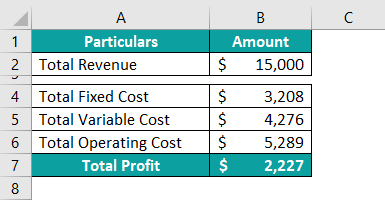Mr. Peter wants to know the profit if the Variable and Operating cost changes to \$5000 and \$6000 respectively. So, let us learn how to use scenario manager in excel.

Step 1: First, open scenario manager from the Data tab and click on the Add button.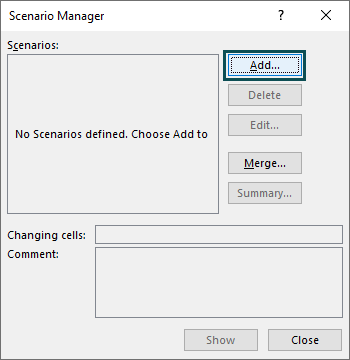Step 2: Next, give a name to the scenario as Alternative 1.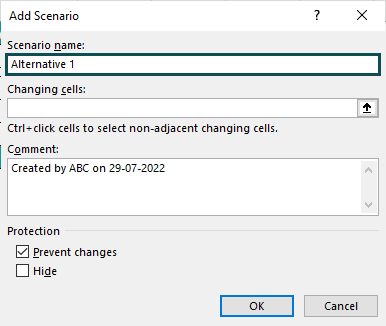Step 3: Then, choose cells B5 & B6 in the Changing cells: box.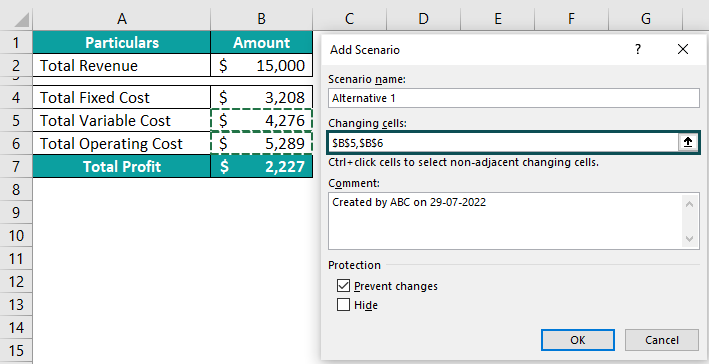Step 4: Next, click on OK and enter the new values.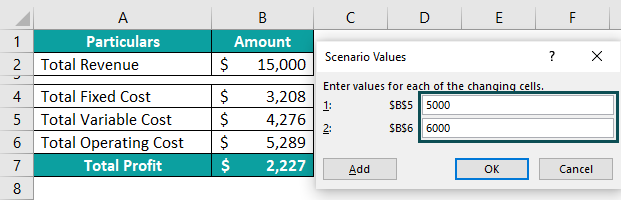Step 5: Finally, click OK, and we will have a scenario ready.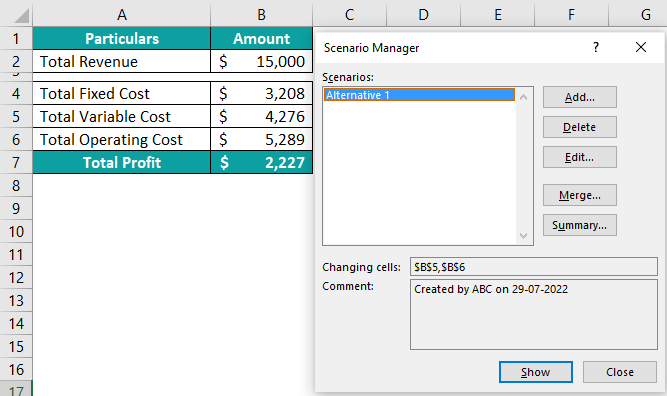Step 6: Now, click on the Show result to see the scenario result.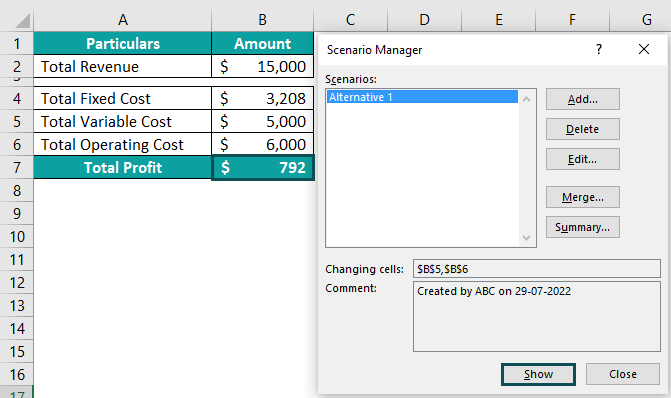With this alternative total profit reduces to \$792.

So, we can use Scenario Manager in excel to make decisions.

Where is Scenario Manager in Excel?

Go to the Data, select the What-If Analysis drop-down list, and click on the Scenario Manager… option.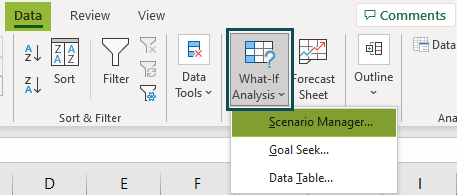How do I run multiple scenarios in Excel with examples?

We can create multiple scenarios in Excel using Scenario manager in excel. But to view them combined, we need to create a summary of scenarios.

Assume we have already created the following 2 scenarios in Excel.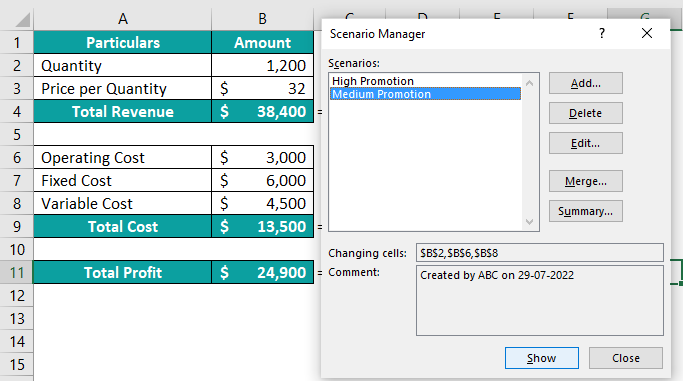In the above window, we need to click on the Summary tab.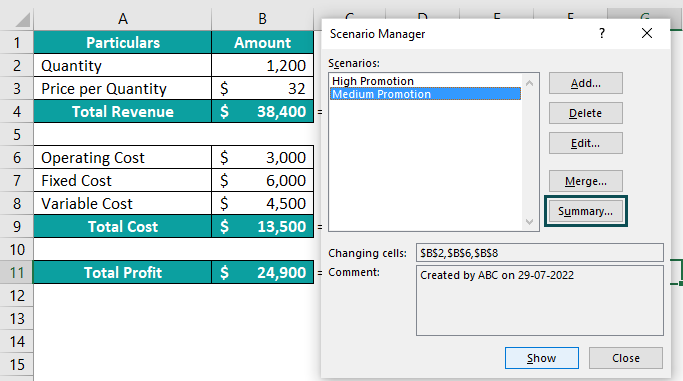After clicking on the summary tab, Excel will ask us to choose the result cells where the output will change. In this case, choose cells B4, B9, and B11.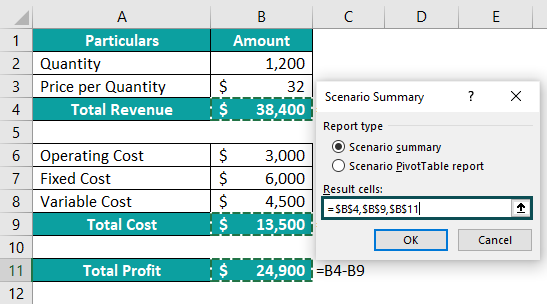Click OK.

Now we have a scenario summary, as shown in the following image.